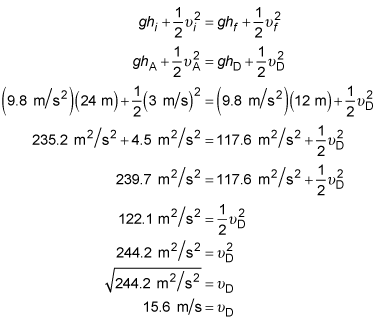##### Physics I: 501 Practice Problems For Dummies (+ Free Online Practice)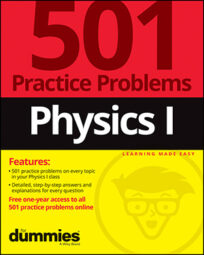In physics, you can find an object's mechanical energy by adding its kinetic energy and its potential energy. For example, you can use the conservation-of-mechanical-energy formula to find the velocity of a cart at different locations on a rollercoaster.

Here are some practice questions that you can try.

## Practice questions

1. A particle has 37.5 joules of kinetic energy and 12.5 joules of gravitational potential energy at one point during its fall from a tree to the ground. An instant before striking the ground, how much mechanical energy — rounded to the nearest joule — does the particle have?

Ignore air resistance.

2. Use the following picture of a cart of mass m moving on a roller coaster, as well as the accompanying information, to answer the following question.

The section of track displayed is frictionless and composed entirely of semicircular pieces of radius r. The letters A through E refer to specific locations along the track.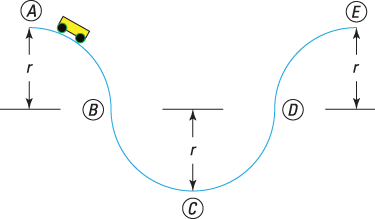If r = 12 meters, and if the cart's velocity at point A is 3 meters per second, what is the cart's velocity at point D? Round your answer to the nearest tenth of a meter per second.

The following are the answers to the practice questions:

1. 50 J

A particle's total mechanical energy is the sum of the particle's kinetic energy and its potential energy. At the first moment mentioned in the problem, the particle has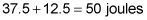of mechanical energy. In the absence of friction (such as air resistance), this amount stays constant throughout the fall. Although the potential energy is 0 on the ground, and therefore all the mechanical energy is in the form of kinetic energy, the particle's total mechanical energy remains 50 joules.

2. 15.6 m/s

First choose a reference location for your gravitational potential energy calculations. (This solution designates point C as the location where h = 0 meters.) Because friction is not present, start with the standard conservation-of-mechanical-energy formula: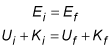where E represents the total mechanical energy, U is the potential energy (entirely gravitational in this problem), and K is the kinetic energy. Then substitute the formulas for kinetic and potential energy: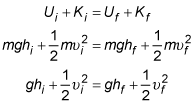where g is the acceleration due to gravity near Earth's surface, h is the height relative to your reference point, and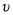is the cart's velocity. The initial location (i) is point A, and the final location (f) is point D. If the height at C is 0 meters, then the height at A is 24 meters,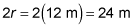and the height at D is 12 meters. Substitute everything you know into the equation to solve for the velocity at point D: